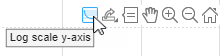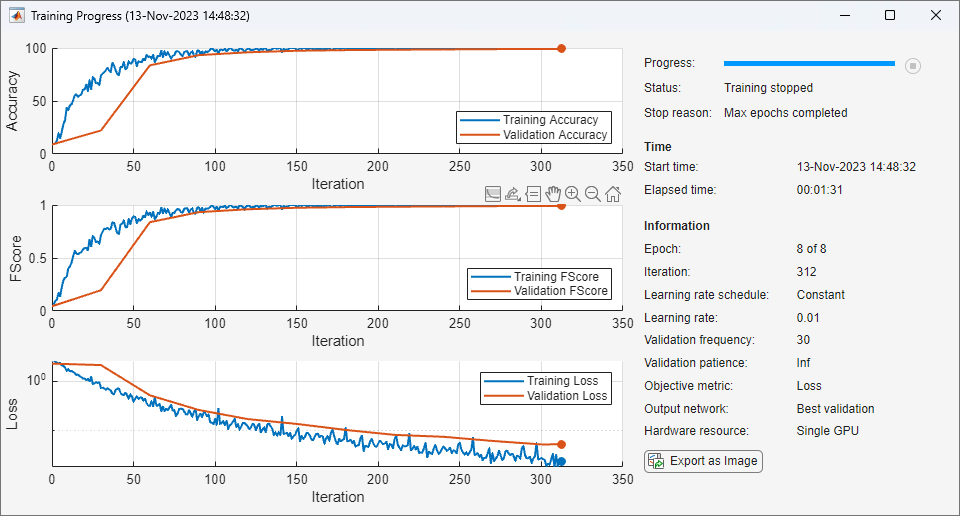# Monitor Deep Learning Training Progress

This example shows how to monitor the training process of deep learning networks.

When you train networks for deep learning, it is often useful to monitor the training progress. By plotting various metrics during training, you can learn how the training is progressing. For example, you can determine if and how quickly the network accuracy is improving, and whether the network is starting to overfit the training data.

When you set the `Plots` training option to `"training-progress"` in `trainingOptions` and start network training, `trainNetwork` creates a figure and displays training metrics at every iteration. Each iteration is an estimation of the gradient and an update of the network parameters. If you specify validation data in `trainingOptions`, then the figure shows validation metrics each time `trainNetwork` validates the network. The figure plots the following:

• Training accuracy — Classification accuracy on each individual mini-batch.

• Smoothed training accuracy — Smoothed training accuracy, obtained by applying a smoothing algorithm to the training accuracy. It is less noisy than the unsmoothed accuracy, making it easier to spot trends.

• Validation accuracy — Classification accuracy on the entire validation set (specified using `trainingOptions`).

• Training loss, smoothed training loss, and validation loss The loss on each mini-batch, its smoothed version, and the loss on the validation set, respectively. If the final layer of your network is a `classificationLayer`, then the loss function is the cross entropy loss. For more information about loss functions for classification and regression problems, see Output Layers.

For regression networks, the figure plots the root mean square error (RMSE) instead of the accuracy.

The figure marks each training Epoch using a shaded background. An epoch is a full pass through the entire data set.

During training, you can stop training and return the current state of the network by clicking the stop button in the top-right corner. For example, you might want to stop training when the accuracy of the network reaches a plateau and it is clear that the accuracy is no longer improving. After you click the stop button, it can take a while for the training to complete. Once training is complete, `trainNetwork` returns the trained network.

When training finishes, view the Results showing the finalized validation accuracy and the reason that training finished. If the `OutputNetwork` training option is `"last-iteration"` (default), the finalized metrics correspond to the last training iteration. If the `OutputNetwork` training option is `"best-validation-loss"`, the finalized metrics correspond to the iteration with the lowest validation loss. The iteration from which the final validation metrics are calculated is labeled Final in the plots.

If your network contains batch normalization layers, then the final validation metrics can be different to the validation metrics evaluated during training. This is because the mean and variance statistics used for batch normalization can be different after training completes. For example, if the `BatchNormalizationStatisics` training option is `"population"`, then after training, the software finalizes the batch normalization statistics by passing through the training data once more and uses the resulting mean and variance. If the `BatchNormalizationStatisics` training option is `"moving"`, then the software approximates the statistics during training using a running estimate and uses the latest values of the statistics.

On the right, view information about the training time and settings. To learn more about training options, see Set Up Parameters and Train Convolutional Neural Network.

To save the training progress plot, click Export Training Plot in the training window. You can save the plot as a PNG, JPEG, TIFF, or PDF file. You can also save the individual plots of loss, accuracy, and root mean squared error using the axes toolbar.### Plot Training Progress During Training

Train a network and plot the training progress during training.

Load the training data, which contains 5000 images of digits. Set aside 1000 of the images for network validation.

```[XTrain,YTrain] = digitTrain4DArrayData; idx = randperm(size(XTrain,4),1000); XValidation = XTrain(:,:,:,idx); XTrain(:,:,:,idx) = []; YValidation = YTrain(idx); YTrain(idx) = [];```

Construct a network to classify the digit image data.

```layers = [ imageInputLayer([28 28 1]) convolution2dLayer(3,8,Padding="same") batchNormalizationLayer reluLayer maxPooling2dLayer(2,Stride=2) convolution2dLayer(3,16,Padding="same") batchNormalizationLayer reluLayer maxPooling2dLayer(2,Stride=2) convolution2dLayer(3,32,Padding="same") batchNormalizationLayer reluLayer fullyConnectedLayer(10) softmaxLayer classificationLayer];```

Specify options for network training. To validate the network at regular intervals during training, specify validation data. Choose the `ValidationFrequency` value so that the network is validated about once per epoch. To plot training progress during training, set the `Plots` training option to `"training-progress"`.

```options = trainingOptions("sgdm", ... MaxEpochs=8, ... ValidationData={XValidation,YValidation}, ... ValidationFrequency=30, ... Verbose=false, ... Plots="training-progress");```

Train the network.

`net = trainNetwork(XTrain,YTrain,layers,options);`